Miscellaneous

Chapter 9 Class 11 Straight Lines
Serial order wise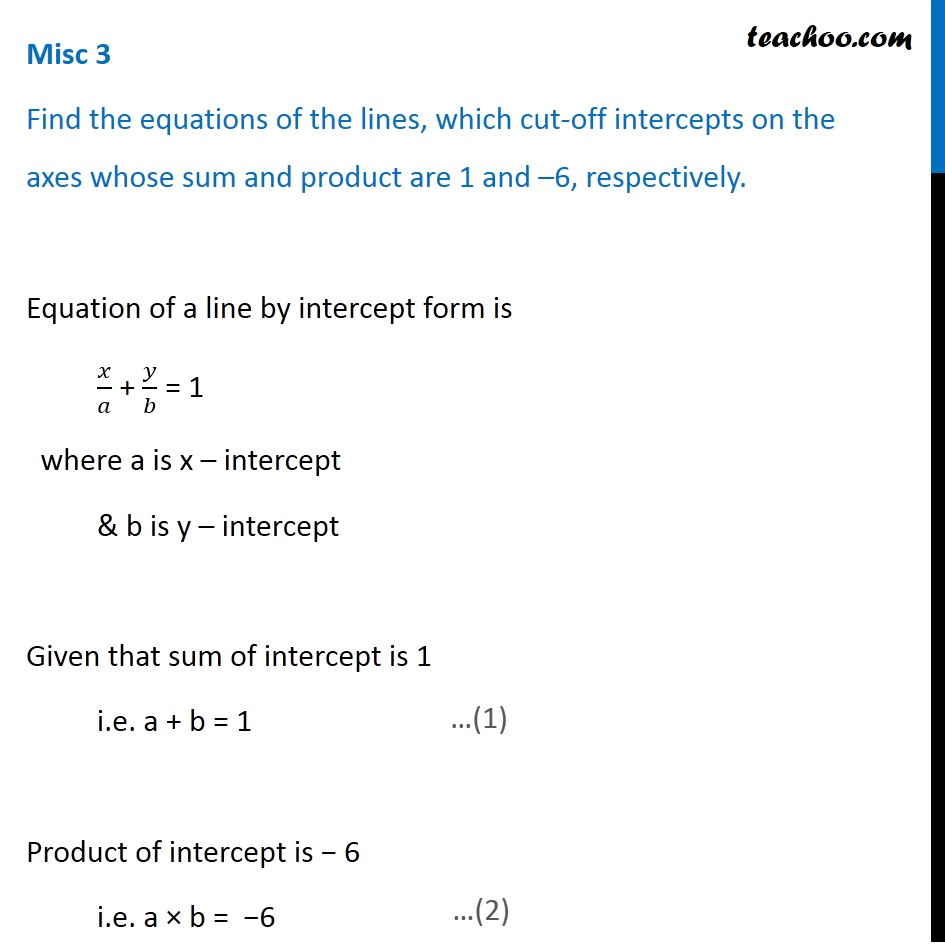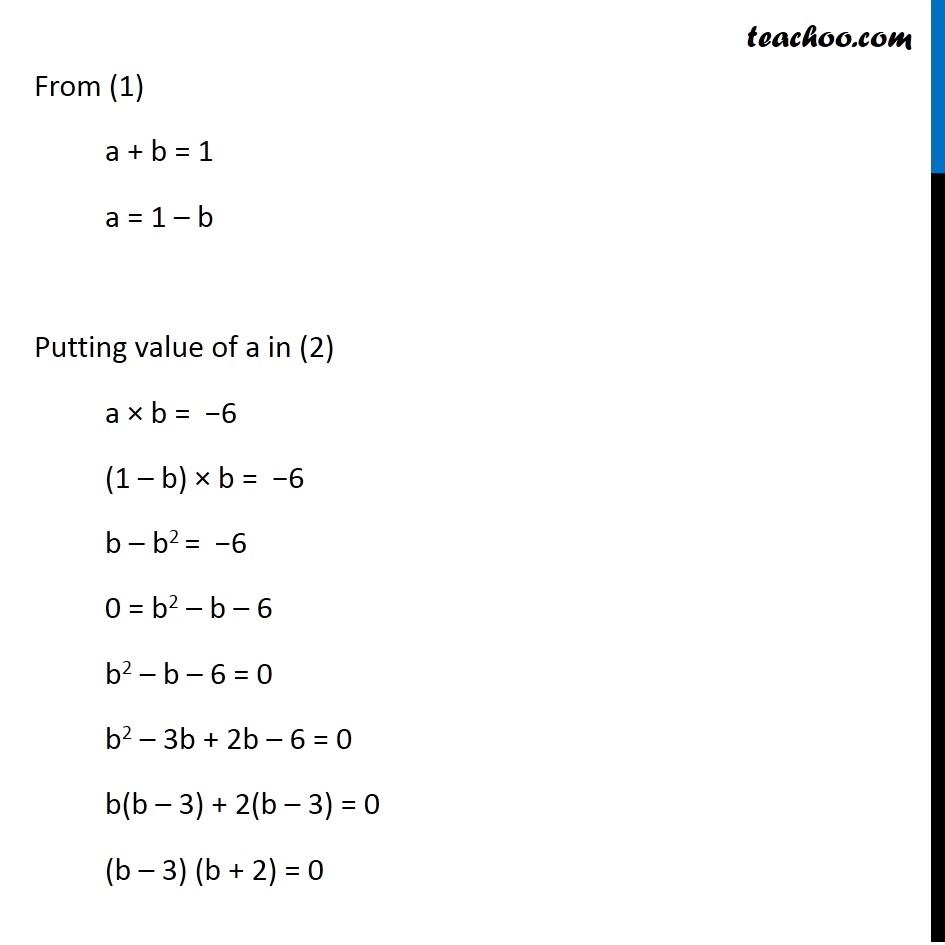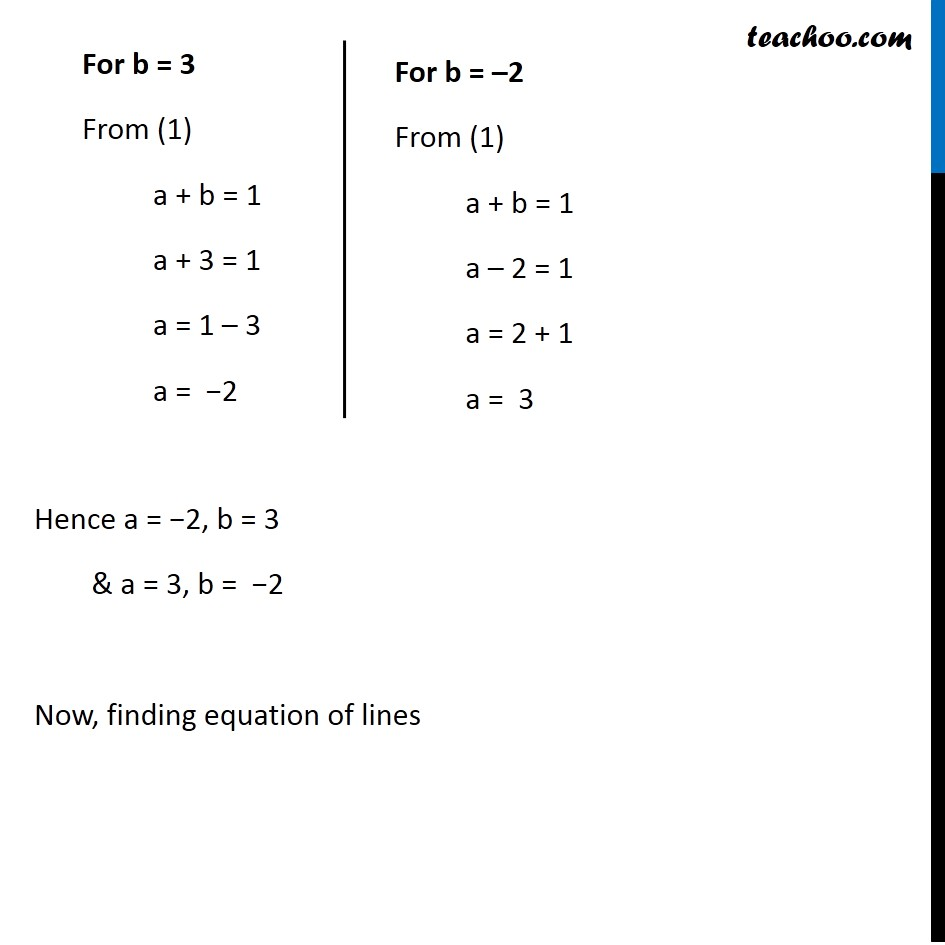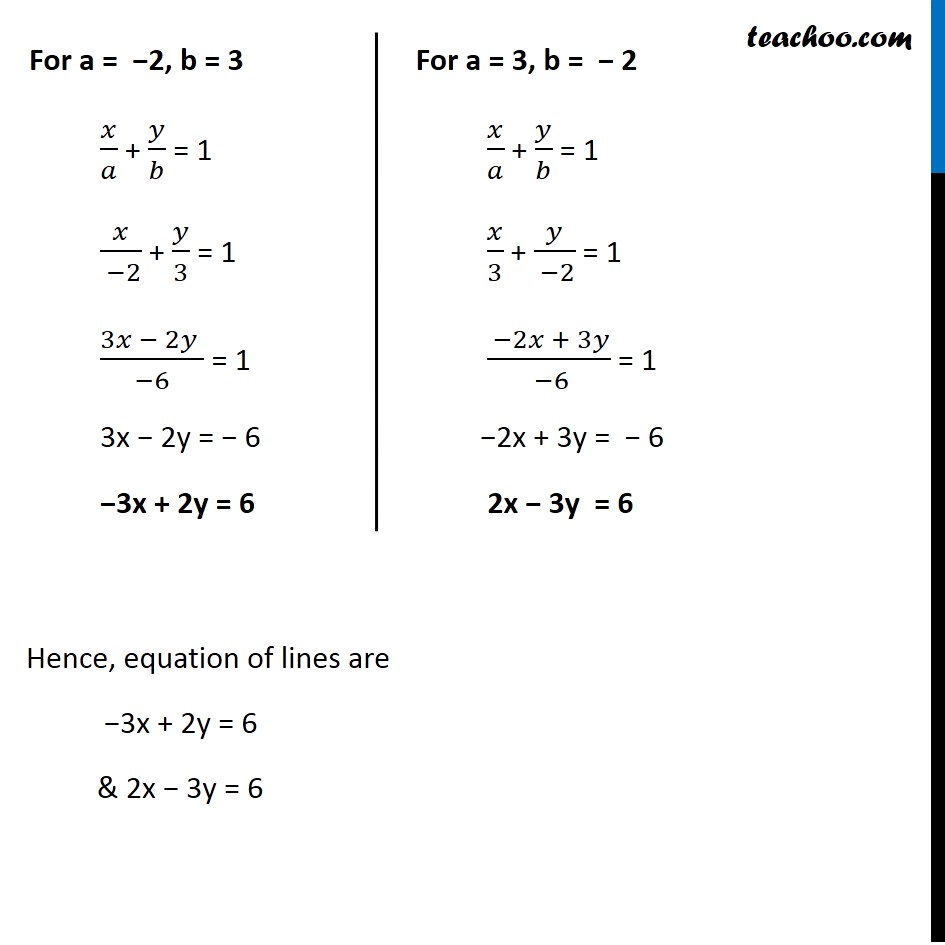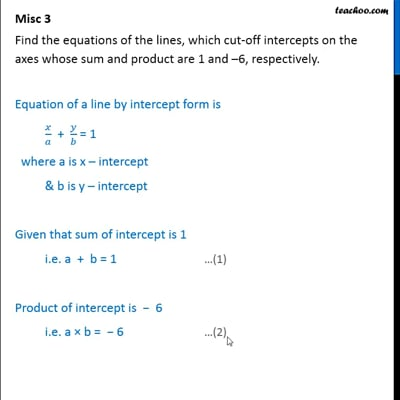This video is only available for Teachoo black users

Learn in your speed, with individual attention - Teachoo Maths 1-on-1 Class

### Transcript

Misc 2 Find the equations of the lines, which cut-off intercepts on the axes whose sum and product are 1 and –6, respectively. Equation of a line by intercept form is 𝑥/𝑎 + 𝑦/𝑏 = 1 where a is x – intercept & b is y – intercept Given that sum of intercept is 1 i.e. a + b = 1 Product of intercept is − 6 i.e. a × b = −6 From (1) a + b = 1 a = 1 – b Putting value of a in (2) a × b = −6 (1 – b) × b = −6 b – b2 = −6 0 = b2 – b – 6 b2 – b – 6 = 0 b2 – 3b + 2b – 6 = 0 b(b – 3) + 2(b – 3) = 0 (b – 3) (b + 2) = 0 So, b = 3, & b = – 2 For b = 3 From (1) a + b = 1 a + 3 = 1 a = 1 – 3 a = −2 For b = –2 From (1) a + b = 1 a – 2 = 1 a = 2 + 1 a = 3 Hence a = −2, b = 3 & a = 3, b = −2 Now, finding equation of lines For a = −2, b = 3 𝑥/𝑎 + 𝑦/𝑏 = 1 𝑥/( −2) + 𝑦/3 = 1 (3𝑥 − 2𝑦 )/( −6 ) = 1 3x − 2y = − 6 −3x + 2y = 6 For a = −2, b = 3 𝑥/𝑎 + 𝑦/𝑏 = 1 𝑥/( −2) + 𝑦/3 = 1 (3𝑥 − 2𝑦 )/( −6 ) = 1 3x − 2y = − 6 −3x + 2y = 6 For a = −2, b = 3 𝑥/𝑎 + 𝑦/𝑏 = 1 𝑥/( −2) + 𝑦/3 = 1 (3𝑥 − 2𝑦 )/( −6 ) = 1 3x − 2y = − 6 −3x + 2y = 6 Hence, equation of lines are −3x + 2y = 6 & 2x − 3y = 6